HETGS Line Profiles

# HETGS Line Profile Simulations

Figure 1a: Fits to a single line, using a Gaussian model, convolved by the instrument response. This is HEG +1 order, Fe XVIII, 14.256 Angstrom line (226 counts). The upper-left frame shows the observed data, and the rest are simulations. Units are counts per bin against wavelength. The red curves are the fits to the simulations. The simulations, shown as black histograms with error bars, are all generated from the same model (upper left pane, red curve). Scales are the same in each panel: 14.145-14.255A, 0-40 counts/bin. The residuals are plotted as \Delta\chi = ( (data-model)/\sigma ).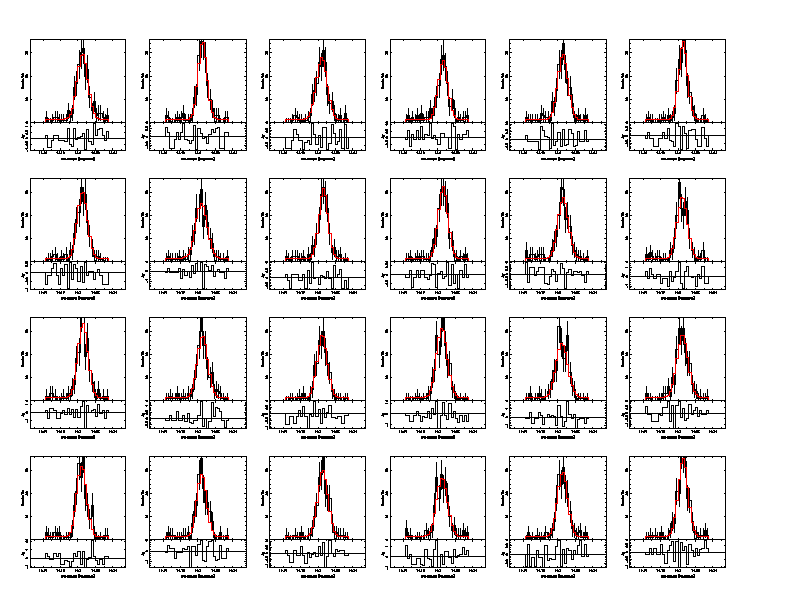Figure 1b: This is the two-parameter chi-squared confidence contour map for flux and wavelength. The solid curves are for the fits to the observation, and are in intervals for 1, 2, and 3 sigma. The best fit to the data is used as the initial values for all the "fakeits", whose best fit parameters are plotted as small circles. For one of these, confidence contours have been computed and are shown as the dashed contours.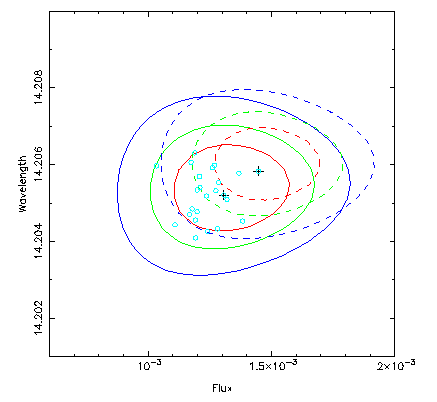Figure 1c: This is the two-parameter chi-squared confidence contour map for flux and Gaussian sigma.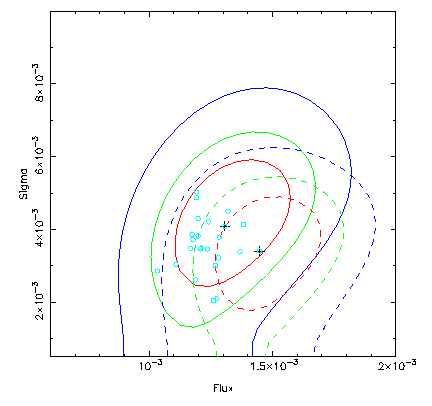Figure 2a: This is similar to Figure 1a except that it is a different spectral region with a nearly-resolved blend, fit using an APED model convolved by the instrument response. This is HEG -1 order, Fe XVII 12.124 + Ne X 12.135 A blend (430 counts). The upper-left frame shows the observed data, and the rest are simulations, as in the previous figure. Scales are 12.10-12.17A and 0-80 counts/bin.Figure 2b: Here are the confidence contours of flux and radial velocity, shown in the same as the previous contour figure. The odd shape of the contours is an artifact of gridding. The model is essentially a delta function, and for small shifts (less than a bin), chi-square does not change. (This could be mitigated by putting both the data and response on a finer grid.)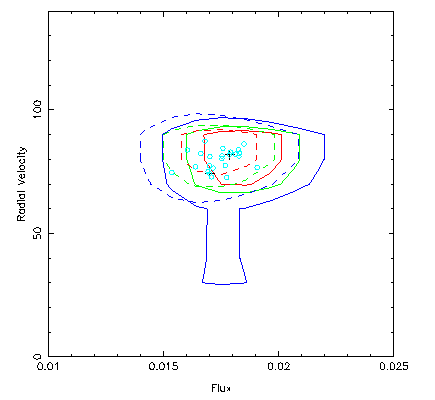Figure 3a: This is like Figure 2a, except that thermal broadening has been included in the APED plasma spectrum evaluation.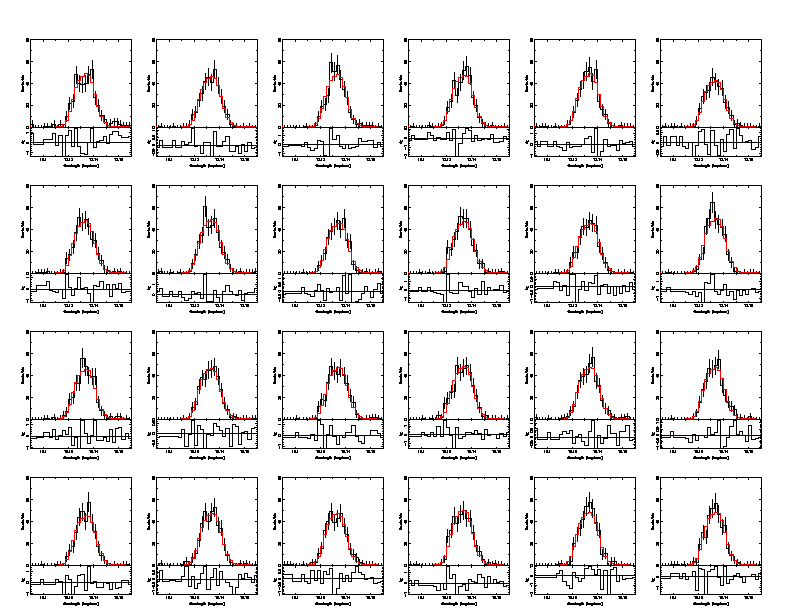Figure 3b: Contour map for thermally-broadened case. Here, since the intrinsic line is broad, the contours are more well behaved.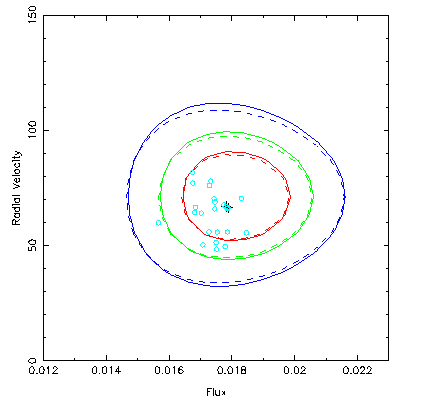Figure 4: This shows the effect of thermal broadening. The red histogram is the model spectrum without broadening, and the green has broadening included. This changes the ratio of the neon and iron components, since they differ greatly in atomic mass.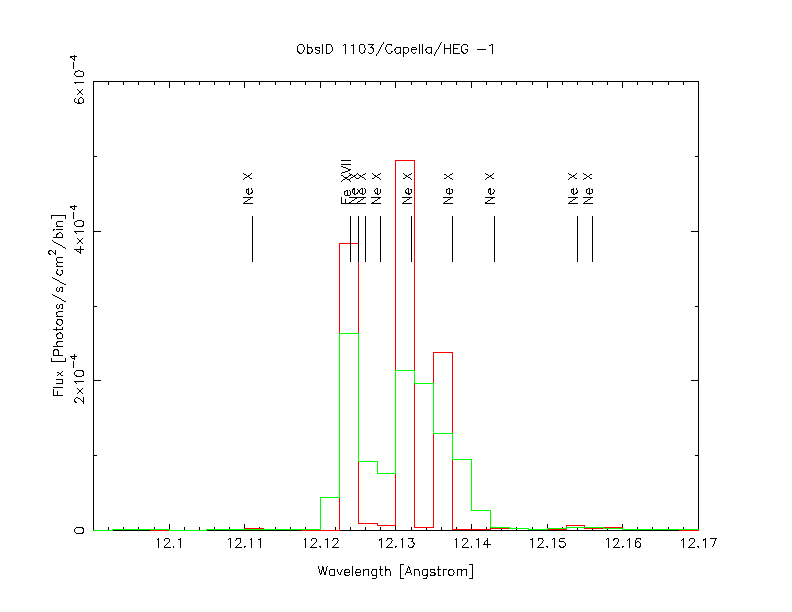(The reader may wish to write an ApJ Letter on the possible detection/absence of thermal broadening in Ne X in Capella.)

Postscript version of the figures:

 Previous: Introduction Next: Script

This page was last updated Jun 6, 2002 by David P. Huenemoerder. To comment on it or the material presented here, send email to dph@space.mit.edu.